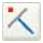# Tekla Structures – Points

Introduction To place an object in a model you may need to pick points. To place an object where no lines or objects intersect, you have the following options to place objects:

•     Use snapping commands.

•     Use construction planes, lines and circles.

•     Create points.

There are many ways to create points in Tekla Structures. Which method is the most convenient at each time, depends on what you have already created in the model and which locations you can easily pick.

When you create points, Tekla Structures always places them according to the work plane coordinate system. Points located in the view plane are yellow and points outside the view plane are red.

Point properties
To view the properties of a point, double-click the point or click Tools > Inquire > Object and select the point.

Phase and ID              Just like any other object, each point has an ID number which is used in log files. Points and

parts have phase numbers. You can filter objects by their phase and ID numbers.

Coordinates               The point information you are most probably interested in is the local (work plane) and global

x, y, and z coordinates of a point. Checking them convinces you of the correct location of the point or some other object related to it.

Creating points

To create points, use the icons on the Points toolbar, or select a command from Modeling > Add Points. The following table lists the commands for creating points and gives a short description of each one:

 Command Icon Description Along Extension of Two Points ■ Creates points as an extension of a line between two picked points. Parallel to Two Points :^ Creates two offset points, parallel to a line, between two picked points. This command also creates construction lines showing offset distances. On Line ‘S Creates points which divide a line.

 Command Icon Description On Plane… Displays the Point Array dialog box and creates a point array relative to the current work plane. Projected Points on LineProjects a picked point onto a picked line. Using Center and Arc Points V. Creates points along an arc. Using Three Arc Points Creates points as an extension of an arc defined by three picked points. Tangent to Circle Creates a point where a circle and a line meet tangentially. At Intersection > Of Two Lines Creates a point at the intersection of two lines. At Intersection > Of Plane and Line •£. Creates a point where a line intersects with a plane. At Intersection > Of Part and Line Creates points where a line intersects with a part surface. At Intersection > Of Circle and Line \d Creates points where a circle and a line intersect. At Intersection > Of Two Part Axes 4 Creates a point where the axes of two parts intersect, and projects the point onto the view plane. At Any Position Creates a point to any position you pick. Bolt Points ■B= Creates points at the bolt center points of the picked bolt groups. Grid Points !# Creates points at the intersections of grid lines on the view plane. 2.5 Construction planes, lines, and circles Construction planes, lines, and circles help you place other objects. For example, you can easily pick the points at intersections of construction lines and circles. The snap priority of construction objects is the same as with the other lines. Construction objects remain in the model when you update or redraw views and windows. They do not appear in drawings. To create construction objects, use the icons on the Points toolbar or select a command on the Modeling menu. The following table lists the commands for creating construction objects and gives a short description of each one:
 Command Icon Description Construction plane Is) Creates a plane. Add Construction Line (2 Creates a construction line between any two picked 3D points. Add Construction Circle Using Center Point and Radius 0 Creates a construction circle in the view plane of the first view picked. Add Construction Circle Using Three Points 0 Creates a construction circle defined by three picked 3D points.
417 views